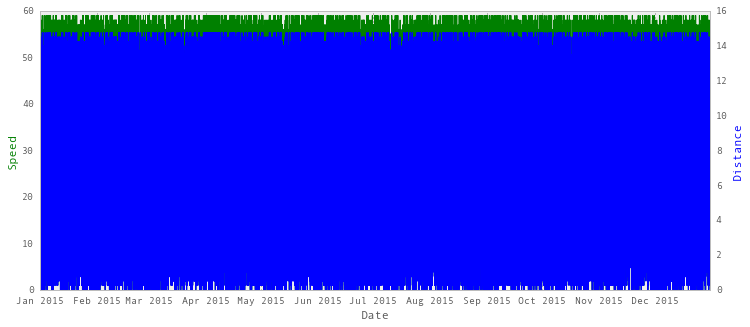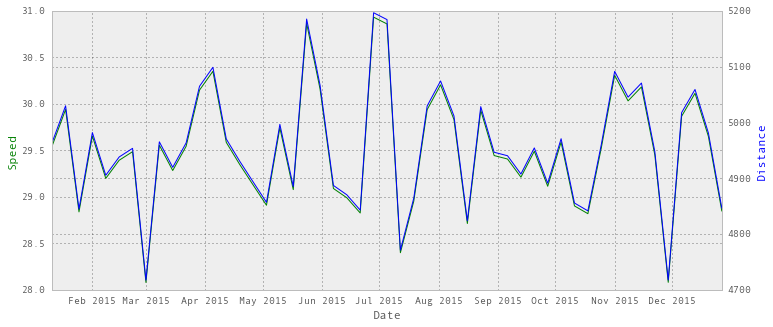## Resampling time series data with pandas

• Post author:
• Post category:Python

# Resampling time series data with pandas

In this post, we’ll be going through an example of resampling time series data using pandas.

We’re going to be tracking a self-driving car at 15 minute periods over a year and creating weekly and yearly summaries.

Let’s start by importing some dependencies:

In :
import pandas as pd
import numpy as np
import matplotlib.pyplot as plt
pd.set_option('display.mpl_style', 'default')
%matplotlib inline


We’ll be tracking this self-driving car that travels at an average speed between 0 and 60 mph, all day long, all year long.

We have the average speed over the fifteen minute period in miles per hour, distance in miles and the cumulative distance travelled.

Our time series is set to be the index of a pandas DataFrame.

In :
range = pd.date_range('2015-01-01', '2015-12-31', freq='15min')
df = pd.DataFrame(index = range)

# Average speed in miles per hour
df['speed'] = np.random.randint(low=0, high=60, size=len(df.index))
# Distance in miles (speed * 0.5 hours)
df['distance'] = df['speed'] * 0.25
# Cumulative distance travelled
df['cumulative_distance'] = df.distance.cumsum()


Let’s take a look at our data:

In :
df.head()

Out:
speed distance cumulative_distance
2015-01-01 00:00:00 9 2.25 2.25
2015-01-01 00:15:00 24 6.00 8.25
2015-01-01 00:30:00 42 10.50 18.75
2015-01-01 00:45:00 22 5.50 24.25
2015-01-01 01:00:00 13 3.25 27.50

Now, let’s try and plot this data:

In :
fig, ax1 = plt.subplots()

ax2 = ax1.twinx()
ax1.plot(df.index, df['speed'], 'g-')
ax2.plot(df.index, df['distance'], 'b-')

ax1.set_xlabel('Date')
ax1.set_ylabel('Speed', color='g')
ax2.set_ylabel('Distance', color='b')

plt.show()
plt.rcParams['figure.figsize'] = 12,5Oh dear… Not very pretty, far too many data points.

The resample method in pandas is similar to its groupby method as you are essentially grouping by a certain time span. You then specify a method of how you would like to resample.

• df.speed.resample() will be used to resample the speed column of our DataFrame
• The 'W' indicates we want to resample by week. At the bottom of this post is a summary of different time frames.
• mean() is used to indicate we want the mean speed during this period.

With distance, we want the sum of the distances over the week to see how far the car travelled over the week, in that case we use sum().

With cumulative distance we just want to take the last value as it’s a running cumulative total, so in that case we use last().

In :
weekly_summary = pd.DataFrame()
weekly_summary['speed'] = df.speed.resample('W').mean()
weekly_summary['distance'] = df.distance.resample('W').sum()
weekly_summary['cumulative_distance'] = df.cumulative_distance.resample('W').last()

#Select only whole weeks
weekly_summary = weekly_summary.truncate(before='2015-01-05', after='2015-12-27')

Out:
speed distance cumulative_distance
2015-01-11 29.549107 4964.25 7738.50
2015-01-18 29.938988 5029.75 12768.25
2015-01-25 28.837798 4844.75 17613.00
2015-02-01 29.653274 4981.75 22594.75
2015-02-08 29.197917 4905.25 27500.00

Now we have weekly summary data. Let’s have a look at our plots now.

In :
fig, ax1 = plt.subplots()

ax2 = ax1.twinx()
ax1.plot(weekly_summary.index, weekly_summary['speed'], 'g-')
ax2.plot(weekly_summary.index, weekly_summary['distance'], 'b-')

ax1.set_xlabel('Date')
ax1.set_ylabel('Speed', color='g')
ax2.set_ylabel('Distance', color='b')

plt.show()
plt.rcParams['figure.figsize'] = 12,5Much better

We can do the same thing for an annual summary:

In :
annual_summary = pd.DataFrame()
# AS is year-start frequency
annual_summary['speed'] = df.speed.resample('AS').mean()
annual_summary['distance'] = df.speed.resample('AS').sum()
annual_summary['cumulative_distance'] = df.cumulative_distance.resample('AS').last()
annual_summary

Out:
speed distance cumulative_distance
2015-01-01 29.489884 1030524 257631.0

### Upsampling data

How about if we wanted 5 minute data from our 15 minute data?

In this case we would want to forward fill our speed data, for this we can use ffil() or pad. Our distance and cumulative_distance column could then be recalculated on these values.

If we wanted to fill on the next value, rather than the previous value, we could use backward fill bfill().

In :
five_minutely_data = pd.DataFrame()
five_minutely_data['speed'] = df.speed.resample('5min').ffill()
# 5 minutes is 1/12 hours
five_minutely_data['distance'] = five_minutely_data['speed'] * (1/float(12))
five_minutely_data['cumulative_distance'] = five_minutely_data.distance.cumsum()

In :
five_minutely_data.head()

Out:
speed distance cumulative_distance
2015-01-01 00:00:00 9 0.75 0.75
2015-01-01 00:05:00 9 0.75 1.50
2015-01-01 00:10:00 9 0.75 2.25
2015-01-01 00:15:00 24 2.00 4.25
2015-01-01 00:20:00 24 2.00 6.25

### Resampling options

pandas comes with many in-built options for resampling, and you can even define your own methods.

In terms of date ranges, the following is a table for common time period options when resampling a time series:

Alias Description
D Calendar day
W Weekly
M Month end
Q Quarter end
A Year end
AS Year start
H Hourly frequency
T, min Minutely frequency
S Secondly frequency
L, ms Millisecond frequency
U, us Microsecond frequency
N, ns Nanosecond frequency

These are some of the common methods you might use for resampling:

Method Description
bfill Backward fill
count Count of values
ffill Forward fill
first First valid data value
last Last valid data value
max Maximum data value
mean Mean of values in time range
median Median of values in time range
min Minimum data value
nunique Number of unique values
ohlc Opening value, highest value, lowest value, closing value